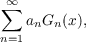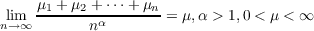#### Vol. 30, No. 3, 1969

 Download this articleFor screen For printingRecent Issues Vol. 325: 1 Vol. 324: 1  2 Vol. 323: 1  2 Vol. 322: 1  2 Vol. 321: 1  2 Vol. 320: 1  2 Vol. 319: 1  2 Vol. 318: 1  2Online Archive Volume: Issue:The Journal Subscriptions Editorial Board Officers Contacts Submission Guidelines Submission Form Policies for Authors ISSN: 1945-5844 (e-only) ISSN: 0030-8730 (print) Special Issues Author Index To Appear Other MSP Journals
Some renewal theorems concerning a sequence of correlated random variables

### G. Sankaranarayanan and C. Suyambulingom

Vol. 30 (1969), No. 3, 785–803
##### Abstract

Consider a sequence {xn},n = 1,2, of random variables. Let Fn(x) be the distribution function of Sn = k=1nxk and Hn(x), the distribution function of Mn = max1knSk. Here we study the asymptotic behaviour of(1.1)

where Gn(x) is to mean either Fn(x) or Hn(x) (so that if a property holds for both Fn(x) and Hn(x) it holds for Gn(x) and conversely) and {an} a suitable positive term sequence, when {xn} form

(i) a sequence of dependent random variables such that the correlation between xi and xj is ρ,ij,i,j = 1,2, , 0 < ρ < 1,E(xi) = μi,i = 1,2, and(1.2)

and

(ii) a sequence of identically distributed random variables with E(xi) = μ,i = 1,2, such that the correlation between xi and xj is ρij = ρ|ij|,i,j = 1,2,,0 < ρ < 1.

Suitable examples are worked out to illustrate the general theory.

Primary: 60.70
##### Milestones